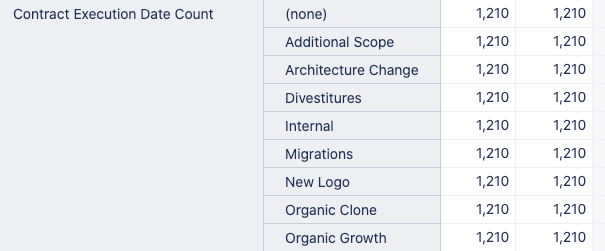# Count issues with filter date or empty

Hi,

I’m trying to get a count of Issues where a custom field of type date is empty or it is greater than 2018.

This is the code I’m trying to use:

``````NonZero(
Count(
Filter(Descendants([Issue].CurrentMember, [Issue].[Issue]),
IsEmpty([Measures].[Issue Contract Execution Date]) OR
(DateCompare(DateWithoutTime([Measures].[Issue Contract Execution Date])
,DateParse('2018-12-31')) > 1)
)
)
)
``````

It is showing some data, but it seems kind of wrong, any ideas why?Issue level calculations pull in any issue imported into the account. You would like to apply the filters to get a correct set of issues for each member (member combinations) used on Rows.

You are using issue properties to represent some logic. Issue properties are great for any issue level calculations. However, they do not validate if the issue is correctly counted for any pages selections on a report.

You would like to use a measure as a filter for any issue level calcualtion if you would like to get some content. The most used measure for this case is Issues created.

Here is an example, how I expanded your formula by adding a filter by measure. I left properties as only filters on the issue set. I used Sum function instead of count and added measure as a counter and it works there as a filter as well:

``````NonZero(
Sum(
Filter(Descendants([Issue].CurrentMember, [Issue].[Issue]),
IsEmpty([Measures].[Issue Contract Execution Date]) OR
(DateCompare(DateWithoutTime([Measures].[Issue Contract Execution Date])
,DateParse('2018-12-31')) > 1)
),
-- counter by measure works as a filter as well:
([Measures].[Issues created],
[Time].CurrentHierarchy.DefaultMember)
)
)
``````

Daina / support@eazybi.com

1 Like

Daina,
The calculation didn’t work as expected, it is filter only the Issues where the ‘Issue Contract Execution Date’ is empty but it’s excluding those where the value is > 2018. So results are very low.
Can you check out the formula and help me to build one that gets those values? Thanks in advance.

Yes, I found an error in the formula. However, it was related to pulling in values for issues with the Contract execution date.

Function DateCompare gives results as -1 (date is before), 0 (date is equal), or 1(date is after). So, the criteria > 1 does not work with DateCompare results.

Here is an updated formula:

``````NonZero(
Sum(
Filter(Descendants([Issue].CurrentMember, [Issue].[Issue]),
IsEmpty([Measures].[Issue Contract Execution Date]) OR
(DateCompare(DateWithoutTime([Measures].[Issue Contract Execution Date])
,DateParse('2018-12-31')) = 1)
),
-- counter by measure works as a filter as well:
([Measures].[Issues created],
[Time].CurrentHierarchy.DefaultMember)
)
)
``````

Daina / support@eazybi.com

1 Like